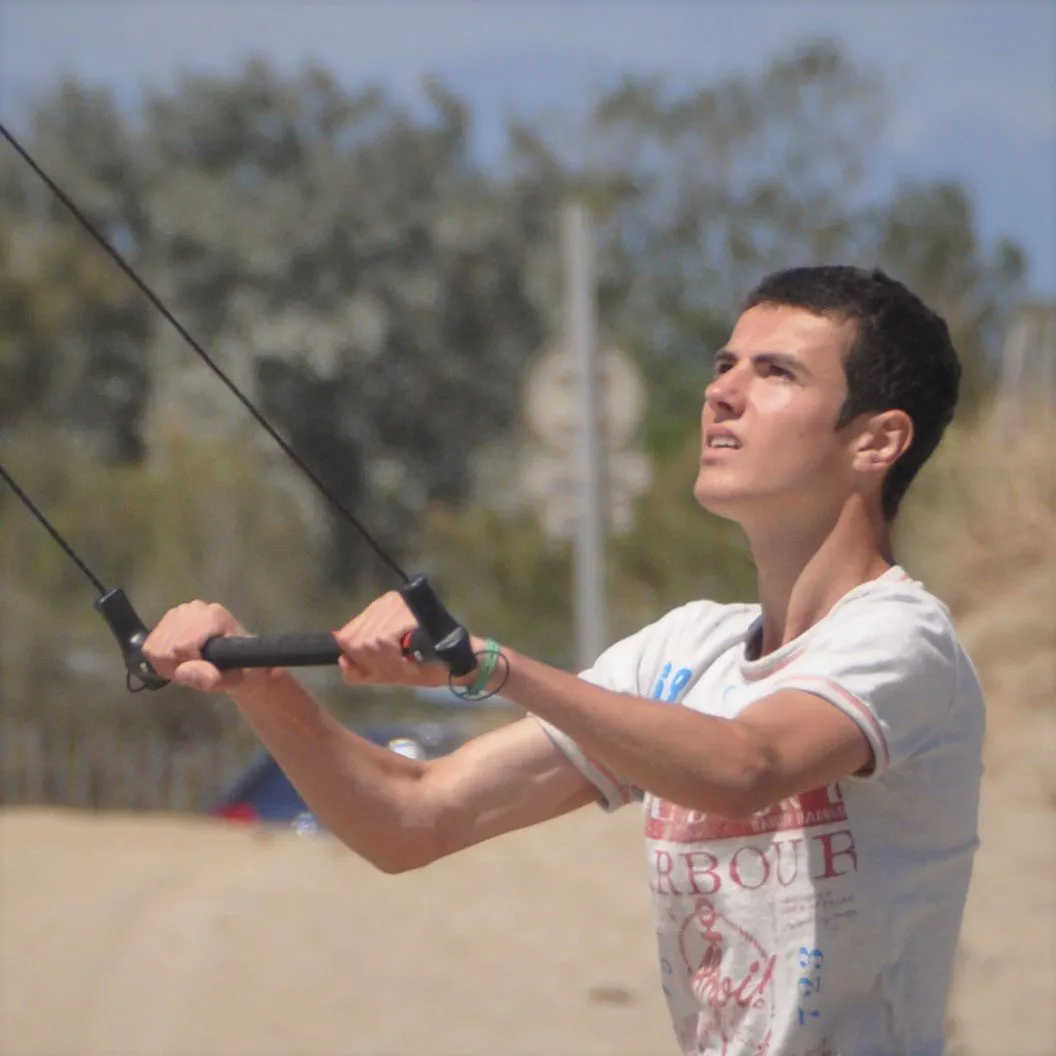Published © GPL3+

# Traffic Light Information System

Traffic Light Information System that lets the driver know at which speed he needs to drive in order to pass the green light.

AdvancedFull instructions provided22,671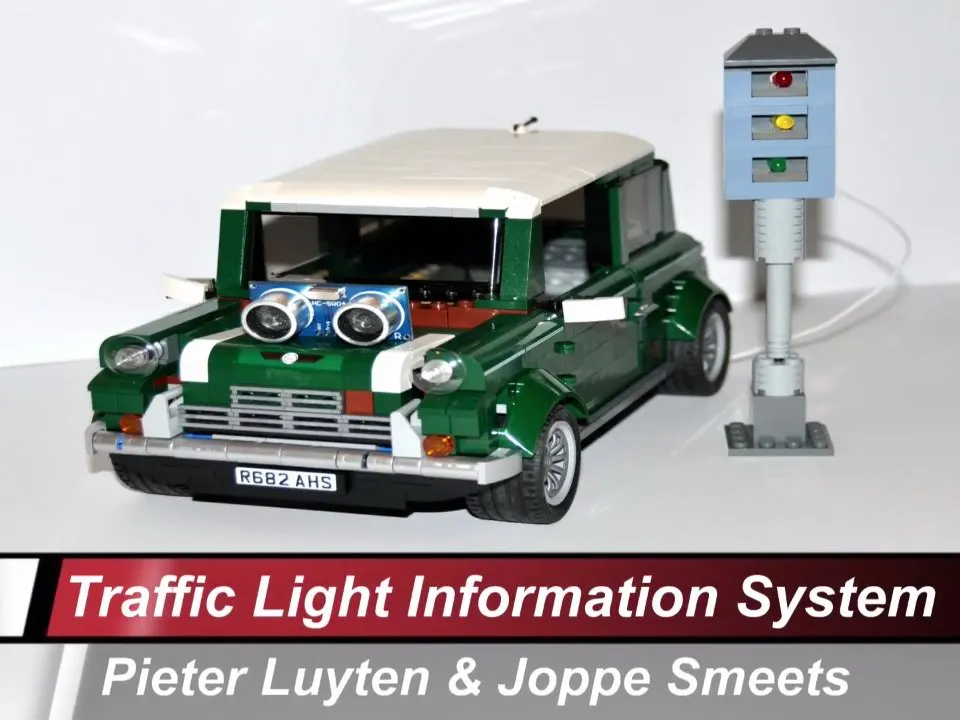## Things used in this project

### Hardware components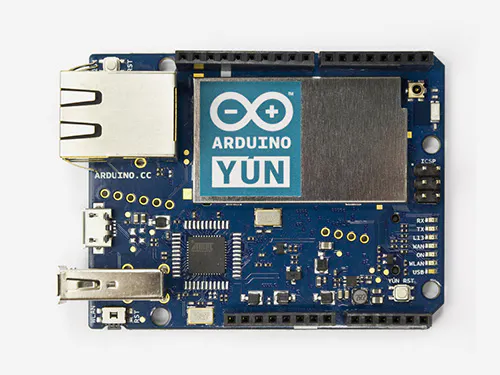Arduino Yun
×1Arduino UNO & Genuino UNO or any other Arduino board
×1Ultrasonic Sensor - HC-SR04 (Generic)
×1
 DS3231 RTC Real Time Clock
×1LED (generic) 1 x Red LED, 1 x Yellow LED, 1 x Green LED
×3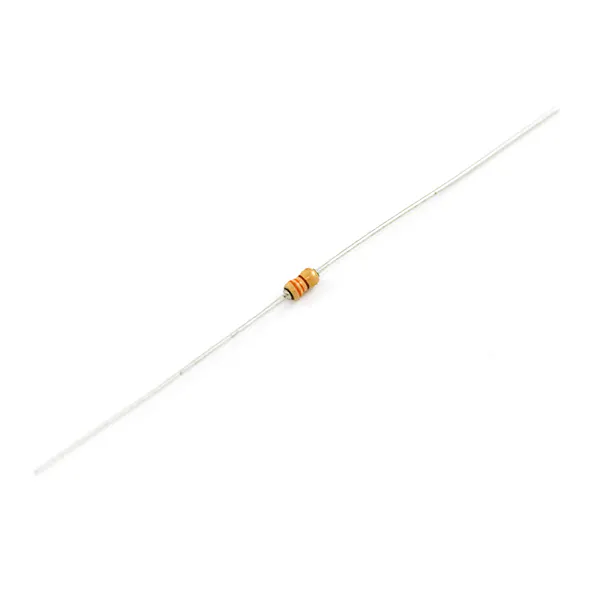Resistor 330 ohm
×3
 433 MHz modules 1 x Transmitter, 1 x Receiver
×1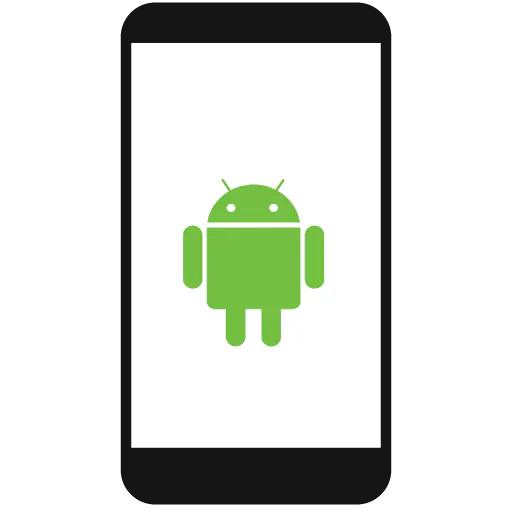Android device
×1
 SD Card
×1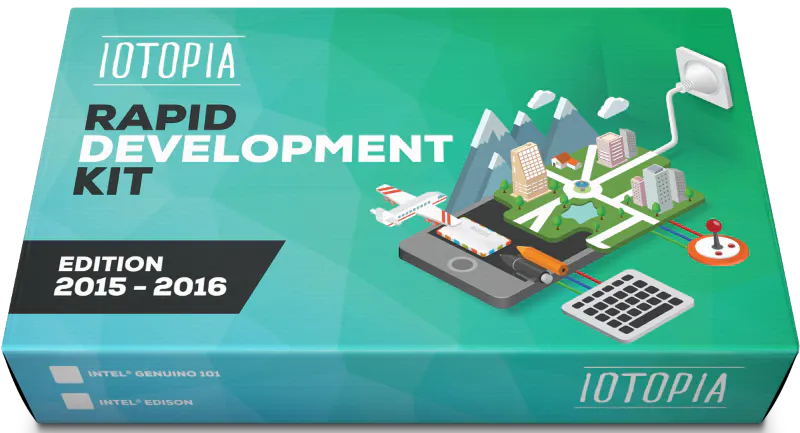AllThingsTalk IOTOPIA Rapid Development kit
×1Jumper wires (generic)
×1

### Software apps and online servicesArduino IDEAndroid Studio
 MySQL database hosting service

Read more

## Schematics

### Schematic: Arduino Transmitter

RF 433MHz module:
tx_pin --> pin 3

HC-SR04 module:
trig_pin --> pin 5
echo_pin --> pin 6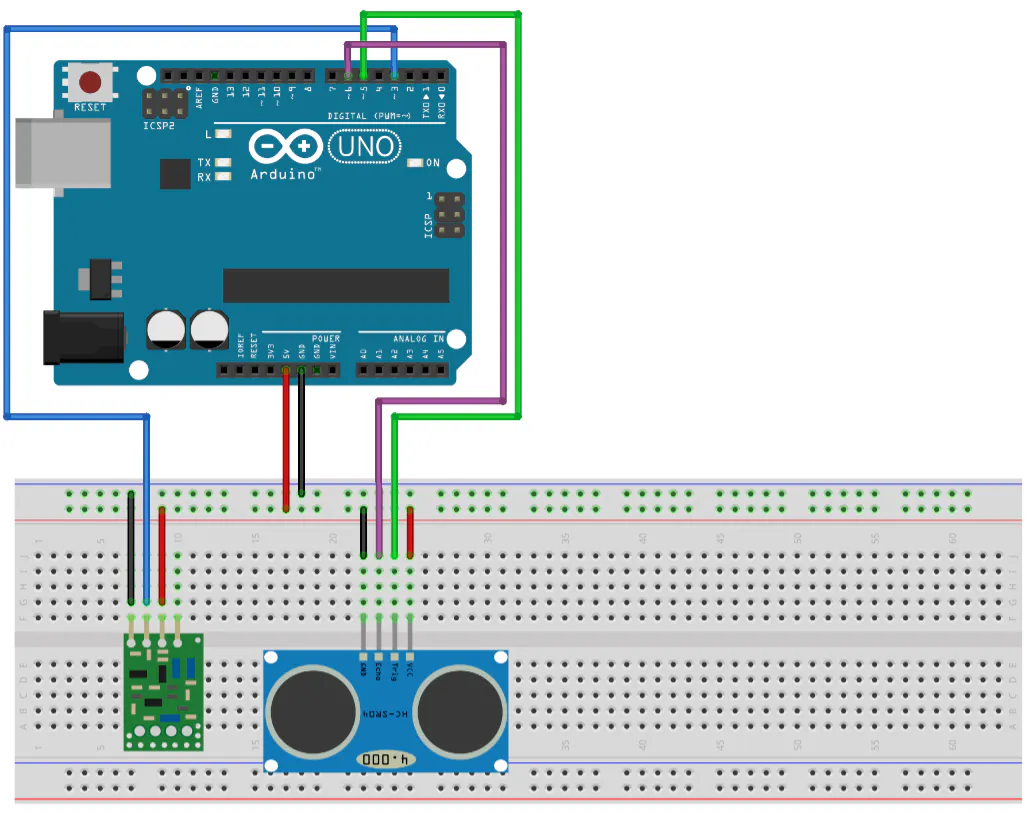### Schematic: Arduino Yun Receiver

RF 433MHz module:
rx_pin --> pin 3

TrafficLight (3 LEDs):
RedLED --> pin 5
OrangeLED --> pin 6
GreenLED --> pin 7

DS3231 (Real-Time-Clock):
SCL --> SCL (pin 3 on Arduino Yun)
SDA --> SDA (pin 2 on Arduino Yun)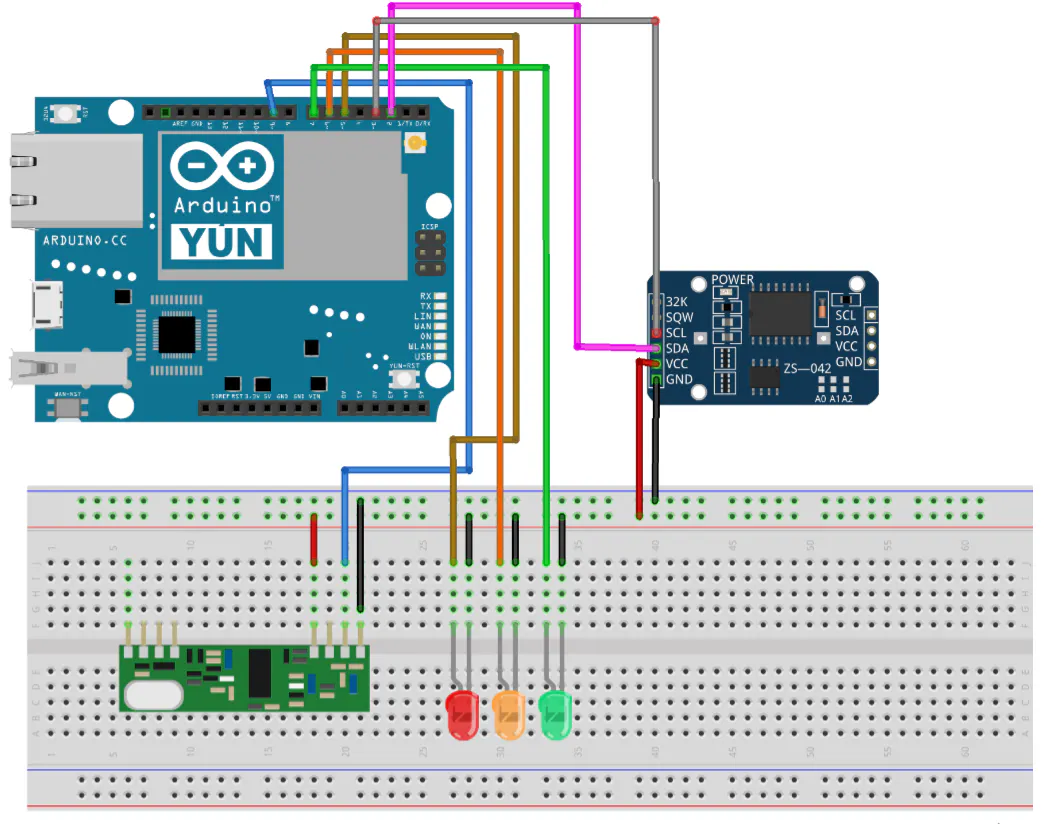## Code

### Arduino Transmitter Code

Arduino
```/*   IOTOPIA - 2016-2017 - Traffic Light Information System (Transmitter)
* by Pieter Luyten & Joppe Smeets
*
* Send distance to another arduino via RF 433MHz modules
*
* RF 433MHz module:
*    tx_pin  --> pin 3
*
* HC-SR04 module:
*    trig_pin  --> pin 5
*    echo_pin  --> pin 6
*
*/

#define tx_pin 3
#define trig_pin 5
#define echo_pin 6

#include <VirtualWire.h> // Library for RF 433 MHz modules

#include "HCSR04.h" // Library for HC-SR04 module
HCSR04 ultrasonic(trig_pin,echo_pin);

int distance;

char CharMsg;

void setup() {
Serial.begin(9600);
vw_setup(2000); // Bits per sec
vw_set_tx_pin(tx_pin);
}

void loop() {

distance = ultrasonic.Ranging(CM); // Measure the distance in cm

while(distance < 0){ // Avoid false readings, measure the distance again
distance = ultrasonic.Ranging(CM);
}

sprintf(CharMsg, "%d,", distance);
vw_send((uint8_t *)CharMsg, strlen(CharMsg)); // Send the distance
vw_wait_tx(); // Wait until the whole message is gone

Serial.print("Distance (cm):  ");
Serial.println(distance);
delay(250);

}
```

### Arduino Receiver & MySQLdb

Arduino
```/*   IOTOPIA - 2016-2017 - Traffic Light Information System  (Receiver + Upload to MySQLdb)
*  by Pieter Luyten & Joppe Smeets
*
*  Receive distance from another arduino via RF
*  Upload traffic light data and distance to MySQL database
*
*  RF 433MHz module:
*     rx_pin  --> pin 3
*
*  TrafficLight (3 LEDs):
*     RedLED  --> pin 5
*     OrangeLED  --> pin 6
*     GreenLED  --> pin 7
*
*  DS3231 (Real-Time-Clock):
*     SCL --> SCL (pin 3 on Arduino Yun)
*     SDA --> SDA (pin 2 on Arduino Yun)
*
*  SD Card - Arduino Yun
*     SD Card (with PHP-file)--> integrated SD slot
*/

#define RedLED  5
#define OrangeLED  6
#define GreenLED  7

#define rx_pin 9

#include <VirtualWire.h> //  RF 433 MHz modules
#include <TimeAlarms.h> //  Alarms
#include <Wire.h> //  I2C communication
#include "RTClib.h" //  Real Time Clock
#include <Process.h> // Run Linux processes on the AR9331

// UnixTime: Time, defined as the number of seconds that have elapsed since 1 January 1970
long UnixTime_green_1; // Next green light (in UnixTime)
long UnixTime_red_1; // Next red light (in UnixTime)
long UnixTime_green_2; // Next but one green light (in UnixTime)
long UnixTime_red_2; // Next but one red light (in UnixTime)
long s_till_orange; // Seconds till orange

RTC_DS3231 rtc;

char StringReceived;

boolean i;

int interval = 5; // in seconds
int distance; // in cm

void setup() {
rtc.begin();
//rtc.adjust(DateTime(F(__DATE__), F(__TIME__)));
Serial.begin(9600);
Bridge.begin(); // Initialize Bridge

vw_setup(2000); // Bits per sec
vw_set_rx_pin(rx_pin);
vw_rx_start();

Alarm.timerRepeat(interval, UpdateTrafficLData); // Update traffic light data (next green/red light ... )
Alarm.timerRepeat(1,PrintCurrentTime); // Print current time every x seconds

pinMode(GreenLED, OUTPUT);
pinMode(OrangeLED, OUTPUT);
pinMode(RedLED, OUTPUT);

digitalWrite(GreenLED, LOW);
digitalWrite(OrangeLED, LOW);
digitalWrite(RedLED, LOW);

Serial.println("Setup finished");
}

void loop() {
Alarm.delay(0); // This delay should be used instead of the normal Arduino delay(),
// for timely processing of alarms and timers. You can pass 0 for minimal delay.

RF_Listen ();
}

void RF_Listen (){
uint8_t buf[VW_MAX_MESSAGE_LEN];
uint8_t buflen = VW_MAX_MESSAGE_LEN;

if (vw_get_message(buf, &buflen)) {
int a;
for (a = 0; a < buflen; a++) {
StringReceived[a] = char(buf[a]);
}

sscanf(StringReceived, "%d", &distance);
MySQL_UpdateDistance();
Serial.print("Distance:  ");
Serial.println(distance);
}
memset( StringReceived, 0, sizeof( StringReceived));
}

void UpdateTrafficLData() {
DateTime now = rtc.now();
if (i == 1) {
UnixTime_green_1 = now.unixtime() + interval + 1;
UnixTime_red_1 = now.unixtime() + 2 * interval + 1;

UnixTime_green_2 = now.unixtime() + 3 * interval + 1;
UnixTime_red_2 = now.unixtime() + 4 * interval + 1;

MySQL_UpdateTrafficLData();

i = 0;
}

else if (i == 0) {
UnixTime_red_1 = now.unixtime() + interval + 1;
UnixTime_green_1 = now.unixtime() + (2 * interval) + 1;

UnixTime_red_2 = now.unixtime()+ 3 * interval + 1;
UnixTime_green_2 = now.unixtime() + 4 * interval + 1;

MySQL_UpdateTrafficLData();

i = 1;
}

Serial.print(" Green_1 at ");
Serial.print(UnixTime_green_1);
Serial.print("   Red_1 at ");
Serial.print(UnixTime_red_1);
Serial.print("   Green_2 at ");
Serial.print(UnixTime_green_2);
Serial.print("   Red_2 at ");
Serial.println(UnixTime_red_2);
}

// Update traffic light data in MySQL database via PHP-script (SD Card)
void MySQL_UpdateTrafficLData() {
long dataArray={UnixTime_green_1, UnixTime_red_1, UnixTime_green_2,UnixTime_red_2};
Process p;
p.begin("/mnt/sda1/MySQL_UpdateTrafficLData.php");
for (int b = 0; b < 4; b++){
p.addParameter(String(dataArray[b]));
}
p.run();

// Read feedback (for debugging)
while (p.available() > 0) {
char c = p.read();
Serial.print(c);
}
// Ensure the last bit of data is sent.
Serial.flush();
}

void MySQL_UpdateDistance(){
Process p;
p.begin("/mnt/sda1/MySQL_UpdateDistance.php");
p.addParameter(String(distance));
p.run();

// Read feedback (for debugging)
while (p.available() > 0) {
char c = p.read();
Serial.print(c);
}
// Ensure the last bit of data is sent.
Serial.flush();
}

void PrintCurrentTime() {
DateTime now = rtc.now();
SetLEDsTrafficL();
Serial.print(now.hour(), DEC);
Serial.print(':');
Serial.print(now.minute(), DEC);
Serial.print(':');
Serial.print(now.second(), DEC);
Serial.print("    ");
Serial.println(now.unixtime());
}

void SetLEDsTrafficL () {
DateTime now = rtc.now();
s_till_orange = UnixTime_red_1 - now.unixtime();

if (i == 0) {
digitalWrite(GreenLED, LOW);
digitalWrite(OrangeLED, LOW);
digitalWrite(RedLED, HIGH);
}

if (i == 1 && s_till_orange <= 3) {
digitalWrite(GreenLED, LOW);
digitalWrite(OrangeLED, HIGH);
digitalWrite(RedLED, LOW);
}

if (i == 1 && s_till_orange > 3) {
digitalWrite(GreenLED, HIGH);
digitalWrite(OrangeLED, LOW);
digitalWrite(RedLED, LOW);
}
}
```

### MySQL_UpdateTrafficLData.php

PHP
```#!/usr/bin/php-cli

<?php
\$UnixTime_green_1 = \$argv;
\$UnixTime_red_1 = \$argv;
\$UnixTime_green_2 = \$argv;
\$UnixTime_red_2 = \$argv;
\$DBServer = 'yourServerName';
\$DBUser   = 'yourUserName';
\$DBPass   = 'yourPassword';
\$DBName   = 'yourDBName';
\$conn = new mysqli(\$DBServer, \$DBUser, \$DBPass, \$DBName);
// check connection
if (\$conn->connect_error) {
trigger_error('Database connection failed: '  . \$conn->connect_error, E_USER_ERROR);
}
\$sql="UPDATE TrafficL_data SET UnixTime_green_1='\$UnixTime_green_1', UnixTime_red_1='\$UnixTime_red_1',UnixTime_green_2='\$UnixTime_green_2', UnixTime_red_2='\$UnixTime_red_2' WHERE id=1";
if(\$conn->query(\$sql) === false) {
trigger_error('Wrong SQL: ' . \$sql . ' Error: ' . \$conn->error, E_USER_ERROR);
}
else{
echo "Data inserted!\n";
}
?>
```

### MySQL_UpdateDistance.php

PHP
```#!/usr/bin/php-cli
<?php
\$Distance = \$argv;
\$DBServer = 'yourServerName';
\$DBUser   = 'yourUserName';
\$DBPass   = 'yourPassword';
\$DBName   = 'yourDBName';
\$conn = new mysqli(\$DBServer, \$DBUser, \$DBPass, \$DBName);
// check connection
if (\$conn->connect_error) {
trigger_error('Database connection failed: '  . \$conn->connect_error, E_USER_ERROR);
}
\$sql="UPDATE TrafficL_data SET Distance='\$Distance'WHERE id=1";

if(\$conn->query(\$sql) === false) {
trigger_error('Wrong SQL: ' . \$sql . ' Error: ' . \$conn->error, E_USER_ERROR);
}
else{
echo "Distance inserted!\n";
}
?>
```

### EchoJSON

PHP
```<?php
define('HOST','yourServerName');
define('USER','yourUserName');
define('PASS','yourPassword');
define('DB','yourDBName');

\$con = mysqli_connect(HOST,USER,PASS,DB);

\$sql = "select * from TrafficL_data";

\$res = mysqli_query(\$con,\$sql);

while(\$row = mysqli_fetch_array(\$res,MYSQLI_BOTH)){
printf('[
{
"TrafficLArray":[
{
"UnixTime_green_1":"%s",
"UnixTime_red_1":"%s",
"UnixTime_green_2":"%s",
"UnixTime_red_2":"%s",
"Distance":"%s"
}
]
}
]', \$row['UnixTime_green_1'], \$row['UnixTime_red_1'],\$row['UnixTime_green_2'],\$row['UnixTime_red_2'],\$row['Distance']);
}

mysqli_close(\$con);

?>
```

### MainActivity.java

Java
```package com.example.xxx.xxx;// change xxx xxx

import android.support.v7.app.AppCompatActivity;
import android.os.Bundle;

import android.util.Log;
import android.widget.TextView;

import com.android.volley.RequestQueue;
import com.android.volley.Response;
import com.android.volley.VolleyError;
import com.android.volley.toolbox.JsonArrayRequest;
import com.android.volley.toolbox.Volley;
import org.json.JSONArray;
import org.json.JSONException;
import org.json.JSONObject;

//import java.text.DateFormat;
import java.util.Date;
import java.util.Timer;
//import java.util.TimerTask;
import java.util.concurrent.Executors;
import java.util.concurrent.Future;
import java.util.concurrent.ScheduledExecutorService;
import java.util.concurrent.TimeUnit;

public class MainActivity extends AppCompatActivity {
// Will show the string "data" that holds the results
TextView results;
//The textview that holds the recommended speed
TextView recSpeed;

// URL of object to be parsed
String JsonURL = "YourURLhere";

// This string will hold the results
String data = "";
// Defining the Volley request queue that handles the URL request concurrently
RequestQueue requestQueue;

//timer object to uppdate the data every second
//Timer timer;

//The speedLimit (cm/s)
private final static double maxSpeed = 18;
//The recommended speed
private double speed;

//number of times in the table
public final static int NUMBER_OF_ENTRIES = 2;
//array with the times when it is green
long[] unixTimesGreen = new long[NUMBER_OF_ENTRIES];
//array with the times when it is red
long[] unixTimesRed = new long[NUMBER_OF_ENTRIES];
//variable which hold the distance
private double distance;

//variables for repeating updateTable
private final ScheduledExecutorService scheduler = Executors.newSingleThreadScheduledExecutor();

//for testing:
private Future<?> timingTask;

public void tick(long milliseconds) {
timingTask = scheduler.scheduleAtFixedRate(new Runnable() {
public void run() {
updateTable();
}
}, 0, milliseconds, TimeUnit.MILLISECONDS);
}

@Override
protected void onCreate(Bundle savedInstanceState) {
super.onCreate(savedInstanceState);
setContentView(R.layout.activity_main);

//textViews to cast results to
results = (TextView) findViewById(R.id.jsonData);

recSpeed = (TextView) findViewById(R.id.recommendedSpeed);

//initialize table
updateTable();

//update app every 100 milliseconds
tick(500);

//for working with a timer object to repeatedly update
/**
timer = new Timer("Timer");
timer.schedule(new UpdateTable(),(long)100,(long)100);
*/

}

//for working with timer object to repeatedly update
/**
private class UpdateTable extends TimerTask{
public void run(){
updateTable();
}
}
*/

private void updateTable(){
//reset String
data = "";
// Creates the Volley request queue
requestQueue = Volley.newRequestQueue(this);

//for testing
/**
// Casts results into the TextView found within the main layout XML with id jsonData
results = (TextView) findViewById(R.id.jsonData);
*/

// Creating the JsonArrayRequest class called arrayreq, passing the required parameters
//JsonURL is the URL to be fetched from
JsonArrayRequest arrayreq = new JsonArrayRequest(JsonURL,
// The second parameter Listener overrides the method onResponse() and passes
//JSONArray as a parameter
new Response.Listener<JSONArray>() {

// Takes the response from the JSON request
@Override
public void onResponse(JSONArray response) {
try {
// Retrieves first JSON object in outer array
JSONObject TrafficLObj = response.getJSONObject(0);
// Retrieves "trafficArry" from the JSON object
JSONArray trafficArry = TrafficLObj.getJSONArray("TrafficLArray");
// Iterates through the JSON Array getting objects and adding them
//to the list view until there are no more objects in trafficArry
for (int i = 0; i < trafficArry.length(); i++) {
//gets each JSON object within the JSON array
JSONObject jsonObject = trafficArry.getJSONObject(i);

//arrays for storing the Strings
String[] green = new String[NUMBER_OF_ENTRIES];
String[] red = new String[NUMBER_OF_ENTRIES];

//String for distance
String distanceTemp = jsonObject.getString("Distance");

distance = (double)Long.parseLong(distanceTemp);

// Retrieves the string
// and converts them into javascript objects
green = jsonObject.getString("UnixTime_green_1");
red = jsonObject.getString("UnixTime_red_1");
green = jsonObject.getString("UnixTime_green_2");
red = jsonObject.getString("UnixTime_red_2");

// Converts Strings retrieved from JSon object to longs
for (int j = 0; j < unixTimesRed.length; j++){
unixTimesGreen[j] = Long.parseLong(green[j]);
unixTimesRed[j] = Long.parseLong(red[j]);
}

//for testing
/**
//create Date Objects to print out readable dates
Date green_1 = new Date(IntUnixTime_green*1000);
Date green_2 = new Date(IntUnixTime_green_2*1000);
Date red_1 = new Date(IntUnixTime_red*1000);
Date red_2 = new Date(IntUnixTime_red_2*1000);

/**
// Adds strings from the current object to the data string
//spacing is included at the end to separate the results from
//one another
data += "\nID " + (i + 1) +
"\nUnixTime green:  " + green_1.toString() +
"\nUnixTime red:  " + red_1.toString() +
"\nUnixTime green_2:  " + green_2.toString() +
"\nUnixTime red_2:  " + red_2.toString()
+
"\nSum = " + (new Date(currentTime)).toString();
*/
}//end for

// Adds the data string to the TextView "results"
//for testing
/**
results.setText(data);
*/

updateTextView();

}//end try

// Try and catch are included to handle any errors due to JSON
catch (JSONException e) {
// If an error occurs, this prints the error to the log
e.printStackTrace();
}//end catch
}//end onResponse
//end response.listener
},
// The final parameter overrides the method onErrorResponse() and passes VolleyError
//as a parameter
new Response.ErrorListener() {
@Override
// Handles errors that occur due to Volley
public void onErrorResponse(VolleyError error) {
Log.e("Volley", "Error");
}
}
);
// Adds the JSON array request "arrayreq" to the request queue
requestQueue.add(arrayreq);
}//end updateTable

private void updateTextView(){
//update time info
StringBuilder builder = new StringBuilder("");

for (int i = 0; i < unixTimesGreen.length; i++){
builder.append("unixTimeGreen");
builder.append(i);
builder.append(": ");
builder.append((new Date(unixTimesGreen[i]*1000)).toString());
builder.append("\n");

builder.append("unixTimeRed");
builder.append(i);
builder.append(": ");
builder.append((new Date(unixTimesRed[i]*1000)).toString());
builder.append("\n");
}
builder.append("distance: " + distance);

//results.setText(builder.toString());

//update speed
speedCalc();
String s = String.format("%.0f",speed);
recSpeed.setText(s);
}

private void speedCalc(){

int interval = 0;
double time = (double)System.currentTimeMillis()/1000;
double diff;

for (int i = 0; i < NUMBER_OF_ENTRIES; i++){
diff = unixTimesRed[interval] - time;
speed = distance/((diff)/1000);
interval++;
}

interval = interval-1;
if(distance/((unixTimesGreen[interval]-time)) > maxSpeed)
speed = maxSpeed;
else
speed = distance/((unixTimesGreen[interval]-time));

speed = speed*5;
}
}
```

### AndroidManifest.xml

XML
```<?xml version="1.0" encoding="utf-8"?>
<manifest xmlns:android="http://schemas.android.com/apk/res/android"
package="com.example.xxx.xxx">// change xxx xxx

<uses-permission android:name="android.permission.INTERNET"/>

<application
android:allowBackup="true"
android:icon="@mipmap/ic_launcher"
android:label="@string/app_name"
android:supportsRtl="true"
android:theme="@style/AppTheme">
<activity android:name=".MainActivity">
<intent-filter>
<action android:name="android.intent.action.MAIN" />

<category android:name="android.intent.category.LAUNCHER" />
</intent-filter>
</activity>
</application>

</manifest>
```

### strings.xml

XML
"NooitRood", meaning: "NeverRed"
```<resources>
<string name="app_name">NooitRood</string>
</resources>
```

### activity_main.xml

XML
```<?xml version="1.0" encoding="utf-8"?>
<LinearLayout xmlns:android="http://schemas.android.com/apk/res/android"
xmlns:tools="http://schemas.android.com/tools"
android:layout_width="match_parent"
android:layout_height="wrap_content"
android:layout_marginLeft="16dp"
android:layout_marginRight="16dp"
tools:context="com.example.xxx.xxx.MainActivity"// change xxx xxx
android:orientation="vertical">

<TextView
android:layout_width="wrap_content"
android:layout_height="wrap_content"
android:layout_gravity="center_horizontal"
android:paddingBottom="10dp"
android:textAppearance="?android:attr/textAppearanceSmall"
android:text="Aanbevolen snelheid:"
android:textSize="20sp"
android:textStyle="bold"
android:id="@+id/jsonData"
android:textColor="#000000" />

<TextView
android:layout_width="900px"
android:layout_height="900px"
android:layout_gravity="center_horizontal"
android:paddingTop="10dp"
android:id="@+id/recommendedSpeed"
android:textSize="120sp"
android:textColor="#000000"
android:textStyle="bold"
android:gravity="center"
android:background="@drawable/gebodsbord"/>

</LinearLayout>
```

## Credits

### Joppe Smeets

1 project • 10 followers

### Pieter Luyten

1 project • 9 followers